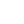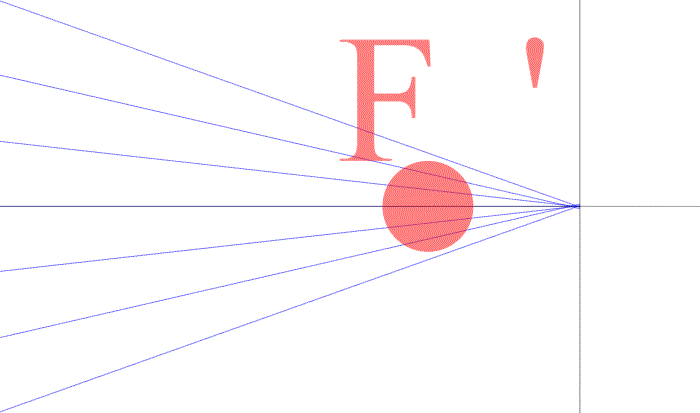----------- Your trusted source for independent sensor data- Photons to Photos------------ Last revised: 2018-02-25 14:00 GMT-5

# ---------------------- Optics Primer - Determining the Focal Length

--------------------------------------------------------- By Bill Claff

In determining magnification I presented the formula:
m = x / f
where m is magnification, x is extension from the image plane, and f is focal length.

We can rearrange this formula as:
f = x / m
So, if we know magnification for a given extension greater than zero we can compute focal length.

For our initial example we have this situation:The extension is 1.72mm and the magnification is 1/30. When we apply the formula we get a focal length of 51.6mm which is quite close to the specification value of 51.61mm.
To avoid paraxial errors the Optical Bench uses a smaller extension in it's calculation and the result for this lens is 51.61mm which matches the specification.

This Optical Bench simulation is exactly the technique I use to measure focal length using a variety of extension tubes and a linear fit to the resulting data.

Measuring focal length using ray tracing will turn out to be important because the patent lens specifications generally only state focal length at infinity and most lenses do not focus solely by extension.
Knowing focal length at other than infinity focus is particularly useful for close-up photographers who want to know what increased magnification will result from a particular extension.